# 基于小波分析的桥梁变形监测数据处理模型Data Processing Model of Bridge Deformation Monitoring Data Based on Wavelet Analysis

DOI: 10.12677/GST.2018.62012, PDF, HTML, XML, 下载: 819  浏览: 1,957

Abstract: Deformation monitoring can analyze and evaluate the safety status of engineering facilities and is an important research content of engineering survey. This article will study the basic principles of wavelet analysis, simulate deformation monitoring data, and establish the data processing models of deformation monitoring. MATLAB software’s wavelet function is used to write the relevant program. The RMSE, SNR and other indicators are used to guide the wavelet decomposition, extract the gross error, and determine the optimal wavelet base and the maximum decomposition scale. At the same time, the wavelet threshold denoising function is studied to determine the best threshold denoising function. Finally, an optimal model is established according to the simulation experiment, and an example of deformation monitoring data is processed, and the processing result is analyzed and the accuracy is evaluated.

1. 引言

2. 研究区概况与研究方法

2.1. 研究区概况

2.2. 研究思路与方法

3. 变形监测数据及分析Figure 1. The accumulative total settlement changes over time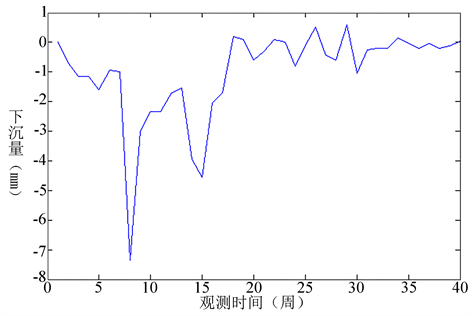Figure 2. The speed of sinking varies with timeTable 1. Bridge deformation monitoring data

4. 变形监测条件确定

4.1. 变形监测数据处理模型

$X=\left\{\begin{array}{l}\mathrm{cos}\left(2\text{π}\cdot 10t\right)\text{\hspace{0.17em}}\text{\hspace{0.17em}}\left(0 (1)

4.2. 确定最大分解尺度

Db小波具有良好的正则性，n值越大，正则性就越好，即光滑性就越好，因此具有良好的分析特性。实验采用db10小波对噪声信号进行不同尺度的分解，图5图6图7图8分别为非平稳信号的不同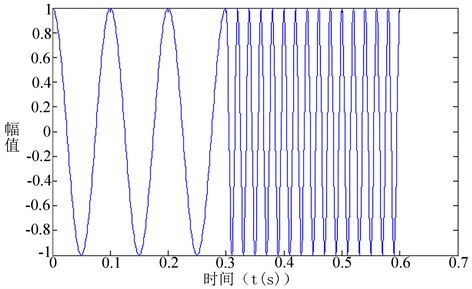Figure 3. Non-stationary signal image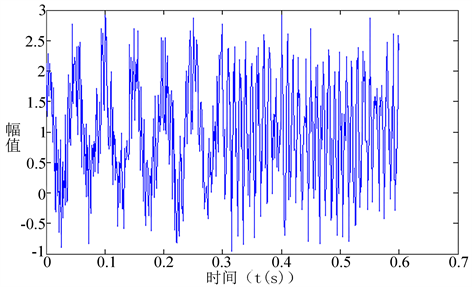Figure 4. The signal after adding the noise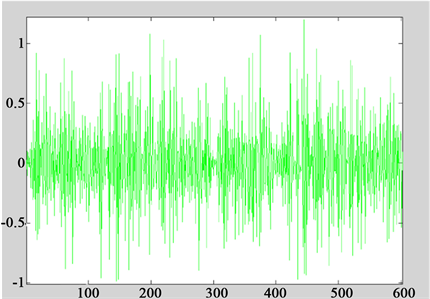Figure 5. 1-level decomposition of non-stationary signals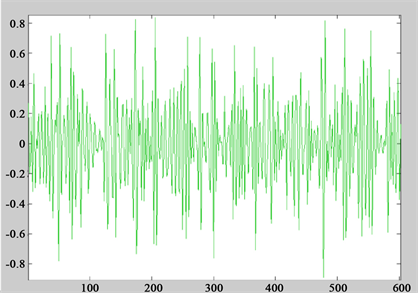Figure 6. 2-level decomposition of non-stationary signal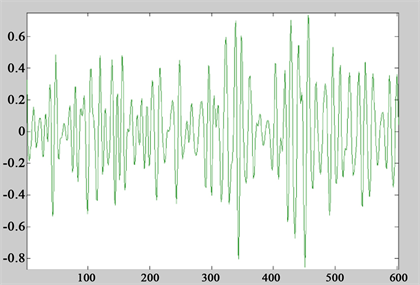Figure 7. 3-level decomposition of non-stationary signals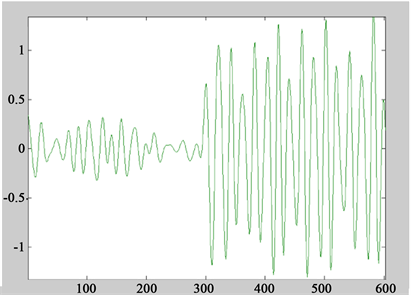Figure 8. 4-level decomposition of non-stationary signals

4.3. 最优小波基的选取

5. 桥梁变形监测处理

5.1. 变形监测数据的粗差探测Figure 9. Raw data image and denoised data image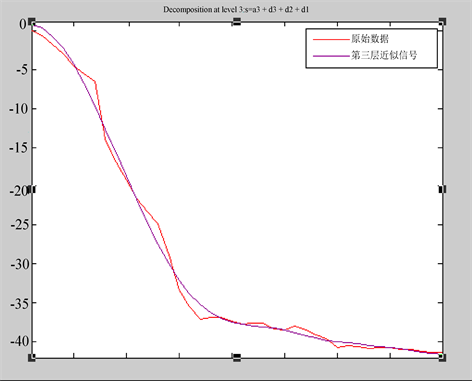Figure 10. The original signal image and the decomposed low frequency signalTable 2. Root mean square error at different levels of decomposition and relative changesTable 3. Signal to noise ratio of all kinds of waveletFigure 11. High-frequency information after the decompositionTable 4. Gross error detection results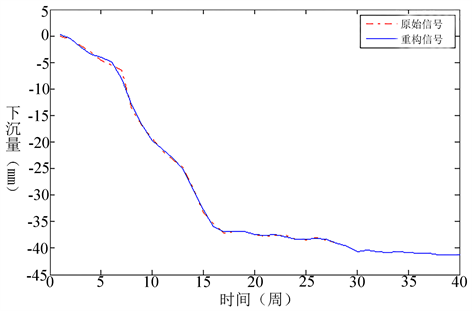Figure 12. The original signal and reconstruction signalTable 5. 4, 5 Interpolation resultsTable 6. 6, 7, 8, 10 Interpolation resultsTable 7. 12, 13, 15 Interpolation results

5.2. 小波阈值去噪

$|{w}_{ij}|\ge \lambda$ 时， $\stackrel{^}{{w}_{ij}}={w}_{ij}$ ；当 $|{w}_{ij}|<\lambda$$\stackrel{^}{{w}_{ij}}=0$ 。该方法为硬阈值去噪法  。采用该方法对信号进行去噪后，再重构信号，去噪后的信号如下图13所示。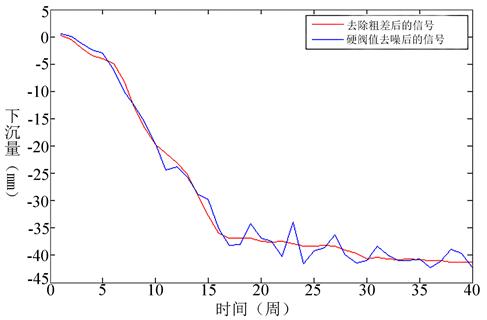Figure 13. The signal after the gross error is removed and the hard threshold denoising.Figure 14. The signal after the gross error is removed and the soft threshold denoising

$\stackrel{^}{{w}_{ij}}=\left\{\begin{array}{l}sign\left({w}_{ij}\right)\left(|{w}_{ij}|-\frac{\lambda }{\mathrm{exp}\left(\frac{|{w}_{ij}|-\lambda }{\lambda }\right)}\right),\text{\hspace{0.17em}}\text{\hspace{0.17em}}|{w}_{ij}|\ge \lambda \\ 0,\text{\hspace{0.17em}}\text{\hspace{0.17em}}\text{\hspace{0.17em}}|{w}_{ij}|<\lambda \end{array}$ (2)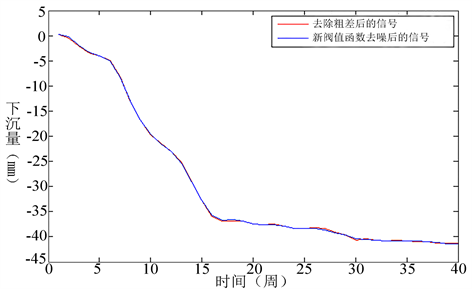Figure 15. Remove the signal after the gross error and the signal after the new threshold denoisingTable 8. Signal-to-noise ratio and mean square error of various threshold functions after denoising

5.3. 去噪结果

6. 结果精度验证Table 9. Deformation monitoring data after denoising (mm)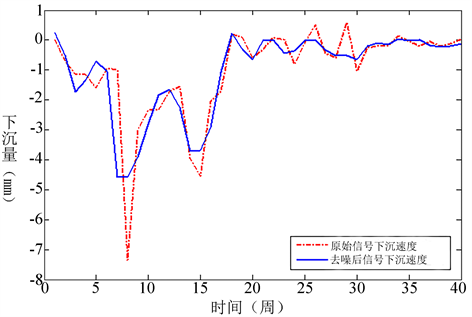Figure 16. The sinking speed of the original signal and the sinking speed of the de-noised signal

7. 结果分析

8. 结论

  王建波. 小波变换在桥梁变形监测数据处理中的应用[D]. 山东科技大学, 2011.  文鸿雁. 小波变换在变形分析建模中的应用[C]//中国科学技术协会. 西部大开发科教先行与可持续发展——中国科协2000年学术年会文集. 中国科学技术协会, 2000: 1.  张勤, 蒋廷臣, 王秀萍. 小波变换在变形监测中的应用研究[J]. 测绘工程, 2005(1): 8-10.  史连艳，杨树兴. 小波滤波在MR/GPS制导系统中的应用[J]. 数据采集与处理, 2006(12): 165-167.  卞继承. 中值滤波和小波变换相结合在信号去噪声中的应用[J]. 科技视界, 2012(28): 292-293.  方杨, 王广兴, 杜玉军, 何薇, 李会梅. 三种粗差检测方法的比较及分析[J]. 测绘通报, 2009(9): 46-61.  刘忠强, 孙昌瑜. 基于变形观测数据粗差探测与修复的小波变换法[J]. 测绘与空间地理信息, 2013(12): 224-226.  肖本林, 李纬, 陈开利. 基于小波变换的GPS变形监测数据处理方法研究[J]. 价值工程, 2010(8): 116-117.  唐桂文, 江恒彪, 左建章. 基于小波阈值去噪理论的监测数据处理方法[J]. 测绘科学, 2009(1): 117-118.  赵瑞珍, 宋国乡, 王红. 小波系数阈值估计的改进模型[J]. 西北工业大学学报, 2001(4): 625-628.  叶裕雷, 戴文战. 一种基于新阈值函数的小波信号去噪方法[J]. 计算机应用, 2006(7): 1617-1619.  王蓓. 基于小波变换的图像去噪算法研究与应用[D]. 延安大学, 2015.  唐桂文, 江恒彪, 左建章. 基于小波阈值去噪理论的监测数据处理方法[J]. 测绘科学, 2009(1): 117-118.  吴兆福, 高飞, 陶庭叶. 小波变换后的噪声信息在大坝变形监测精度评定中的应用[J]. 地球科学进展, 2008(6): 590-594.  黄声享, 刘经南. GPS变形监测系统中消除噪声的一种有效方法[J]. 测绘学报, 2002(2): 104-107.  陈彦. 小波变换的在形变监测数据处理中的应用研究[J]. 四川地质学报, 2013(S1): 197-200.  万程辉, 欧阳平. 大坝变形监测数据的小波分析处理方法[J]. 北京测绘, 2010(1): 32-34.